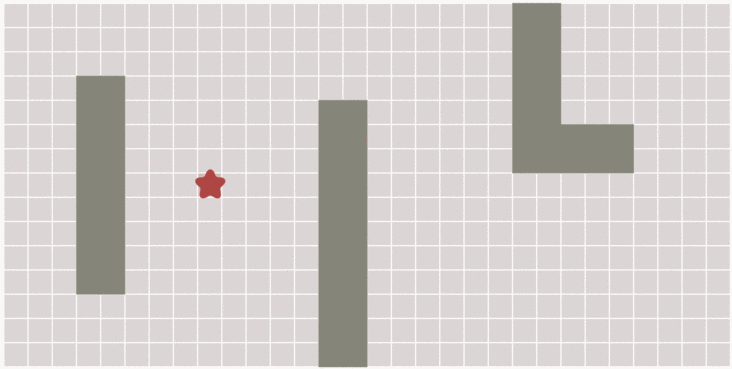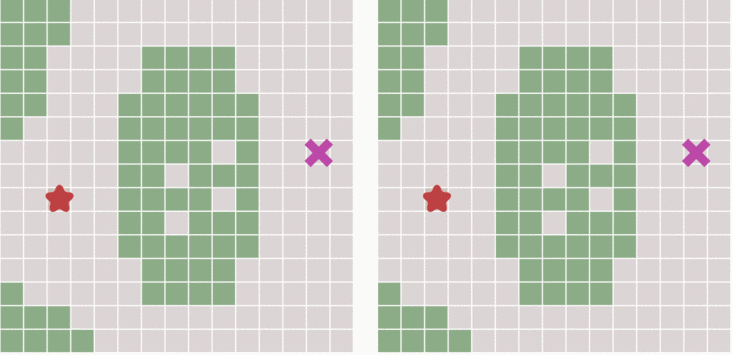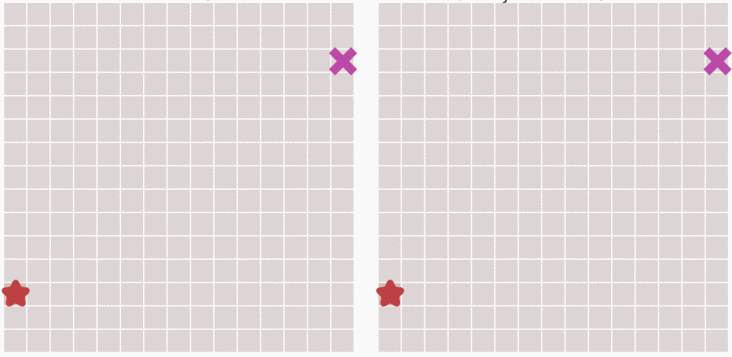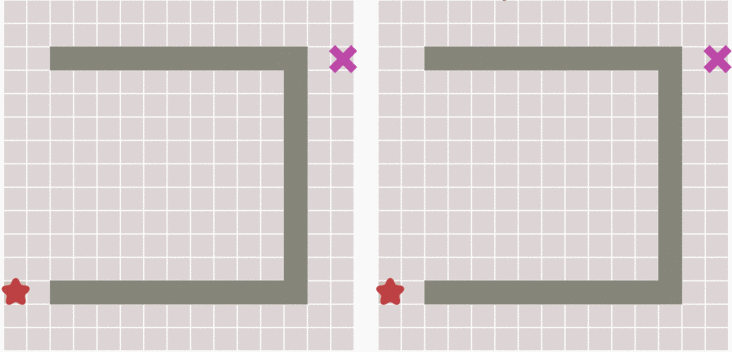# 前言

A 星搜索算法发表于 1968 年属于比较老、成熟的算法，由 Stanford 研究院的 Peter Hart, Nils Nilsson 以及 Bertram Raphael 发表。介绍 A 星算法本来应该先了解 A 算法，但这里先不说 A 算法，先来感性的了解一下跟它有关的其他算法。

## 广度优先搜索算法## Dijkstra 算法## 最佳优先搜索算法Dijkstra 算法是根据距离起点的代价来计算下一步该选择哪一个格子的，那么如果我们最开始就预先估计下一步中的所有格子哪一个离终点更近，这样也能更快到达终点。如上图，所示每次选取都选择离终点最近的格子，然后再遍历这个格子附近的格子哪个离终点更近（一般使用 $$h(n)$$ 表示第 n 个格子到终点的代价）这种算法称之为最佳优先算法。# A * 搜索算法

A 星算法则是整合上述的优缺点，首先来明白几个概念：

$$g(n)$$ 代表移动到第 n 个方格时距离起点的代价。$$g(n)$$ 的大小肯定是跟之前的 n-1 个格子的选择有关的，但是每次选择肯定都是选择代价最小的格子（如果仅用 $$g(n)$$ 来衡量）。

$$h(n)$$ 代表移动到第 n 个方格时距离终点的估计代价（往往我们并不完全知道这个格子距离终点需要多少代价）。$$h(n)$$ 的大小范围（也就是第 n 步能选择的范围）当然也是跟之前的 n-1 个格子的选择有关的，但是每次选择肯定都是选择代价最小的格子（如果仅用 $$h(n)$$ 来衡量）。

$$f(n)$$ 是整体的估价函数，一般 $$f(n) = g(n) + h(n)$$

## 算法细节

• 首先将初始的节点 $$s_0$$（可以理解为起点的格子）放入 open 表中，因为没有其他选择-_-。此时 $$f(s_0) = g(s_0) + h(s_0)$$
• 如果 open 表为空，表示上一步没有留下可以被选择的节点，则问题无解，退出搜索；
• 把 open 表的第一个节点取出来放入 closed 表，并记该节点为 $$n$$（即第 n 步选择的节点）。
• 考察节点 n 是否为目标节点，若是，找到问题解，退出。
• 若节点 n 不可以扩展，则转到第二步；
• 扩展节点 n（即查看这个格子周围的格子），生成子节点 $$n_i \ (i=1,2,3,\dots)$$，首先这些子节点得不在 closed 表中，也不再 open 表中，然后放入 open 表中。计算每个子节点的估价值 $$f(n_i)$$，并按估价值从小到大的顺序排好。
• 转至第二步。

## A * 算法的一些性质

A * 算法对上述进行了限制：

$$g(n)$$ 是对 $$g^*(n)$$ 的估计，且有 $$g(n) \gt g^*(n) \ge 0$$
$$h(n)$$ 会被设计为 $$h^*(n)$$ 的下界，且有 $$h^*(n) \gt h^(n)$$

### 可纳性

\begin{aligned} f(n_1) &= g(n_1) + h(n_1) \\ &= g^*(n) + h(n_1) \\ &\le g^*(n) + h^*(n) = f^*(n_1) = f^*(s_0) = f(s_0) \end{aligned}

### 最优性

A 星算法的搜索效率其实极大取决于 $$h(n)$$，因为 $$h(n) \le h^*(n)$$ 的，所以它越大可以扩展的节点就越少，搜索的效率就越高。此外，关于 $$h(n)$$ 的设计还需要满足：

$h(n_i) + g(n_i) \le h(n_j) + g(n_j)$

Tags: